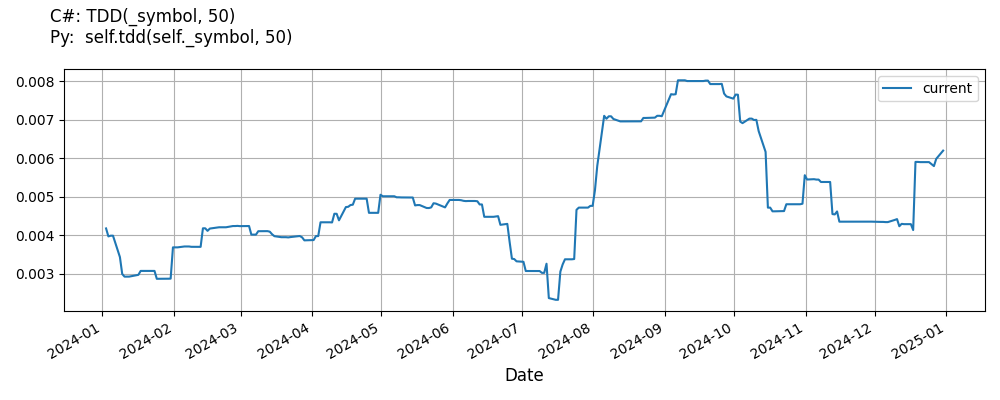# Supported Indicators

## Target Downside Deviation

### Introduction

This indicator computes the n-period target downside deviation. The target downside deviation is defined as the root-mean-square, or RMS, of the deviations of the realized return’s underperformance from the target return where all returns above the target return are treated as underperformance of 0. Reference: https://www.cmegroup.com/education/files/rr-sortino-a-sharper-ratio.pdf

To view the implementation of this indicator, see the LEAN GitHub repository.

### Using TDD Indicator

To create an automatic indicators for TargetDownsideDeviation, call the TDD helper method from the QCAlgorithm class. The TDD method creates a TargetDownsideDeviation object, hooks it up for automatic updates, and returns it so you can used it in your algorithm. In most cases, you should call the helper method in the Initialize method.

public class TargetDownsideDeviationAlgorithm : QCAlgorithm
{
private Symbol _symbol;
private TargetDownsideDeviation _tdd;

public override void Initialize()
{
_tdd = TDD(_symbol, 50);
}

public override void OnData(Slice data)
{
{
// The current value of _tdd is represented by itself (_tdd)
// or _tdd.Current.Value
Plot("TargetDownsideDeviation", "tdd", _tdd);

}
}
}
class TargetDownsideDeviationAlgorithm(QCAlgorithm):
def Initialize(self) -> None:
self.tdd = self.TDD(self.symbol, 50)

def OnData(self, slice: Slice) -> None:
# The current value of self.tdd is represented by self.tdd.Current.Value
self.Plot("TargetDownsideDeviation", "tdd", self.tdd.Current.Value)



The following reference table describes the TDD method:

### TDD()1/1

            TargetDownsideDeviation QuantConnect.Algorithm.QCAlgorithm.TDD (
Symbol                           symbol,
Int32                            period,
*Double                          minimumAcceptableReturn,
*Nullable<Resolution>      resolution,
*Func<IBaseData, Decimal>  selector
)


Creates a new TargetDownsideDeviation indicator. The target downside deviation is defined as the root-mean-square, or RMS, of the deviations of the realized return’s underperformance from the target return where all returns above the target return are treated as underperformance of 0.

If you don't provide a resolution, it defaults to the security resolution. If you provide a resolution, it must be greater than or equal to the resolution of the security. For instance, if you subscribe to hourly data for a security, you should update its indicator with data that spans 1 hour or longer.

You can manually create a TargetDownsideDeviation indicator, so it doesn't automatically update. Manual indicators let you update their values with any data you choose.

Updating your indicator manually enables you to control when the indicator is updated and what data you use to update it. To manually update the indicator, call the Update method with time/number pair, or an IndicatorDataPoint. The indicator will only be ready after you prime it with enough data.

public class TargetDownsideDeviationAlgorithm : QCAlgorithm
{
private Symbol _symbol;
private TargetDownsideDeviation _tdd;

public override void Initialize()
{
_tdd = new TargetDownsideDeviation(TargetDownsideDeviation(50), RateOfChange(1));
}

public override void OnData(Slice data)
{
if (data.Bars.TryGeValue(_symbol, out var bar))
{
_tdd.Update(bar.EndTime, bar.Close);
}

{
// The current value of _tdd is represented by itself (_tdd)
// or _tdd.Current.Value
Plot("TargetDownsideDeviation", "tdd", _tdd);

}
}
}
class TargetDownsideDeviationAlgorithm(QCAlgorithm):
def Initialize(self) -> None:
self.tdd = TargetDownsideDeviation(TargetDownsideDeviation(50), RateOfChange(1))

def OnData(self, slice: Slice) -> None:
bar = slice.Bars.get(self.symbol)
if bar:
self.tdd.Update(bar.EndTime, bar.Close)

# The current value of self.tdd is represented by self.tdd.Current.Value
self.Plot("TargetDownsideDeviation", "tdd", self.tdd.Current.Value)



To register a manual indicator for automatic updates with the security data, call the RegisterIndicator method.

public class TargetDownsideDeviationAlgorithm : QCAlgorithm
{
private Symbol _symbol;
private TargetDownsideDeviation _tdd;

public override void Initialize()
{
_tdd = new TargetDownsideDeviation(TargetDownsideDeviation(50), RateOfChange(1));
RegisterIndicator(_symbol, _tdd, Resolution.Daily);
}

public override void OnData(Slice data)
{
{
// The current value of _tdd is represented by itself (_tdd)
// or _tdd.Current.Value
Plot("TargetDownsideDeviation", "tdd", _tdd);

}
}
}
class TargetDownsideDeviationAlgorithm(QCAlgorithm):
def Initialize(self) -> None:
self.tdd = TargetDownsideDeviation(TargetDownsideDeviation(50), RateOfChange(1))
self.RegisterIndicator(self.symbol, self.tdd, Resolution.Daily)

def OnData(self, slice: Slice) -> None:
# The current value of self.tdd is represented by self.tdd.Current.Value
self.Plot("TargetDownsideDeviation", "tdd", self.tdd.Current.Value)



The following reference table describes the TargetDownsideDeviation constructor:

### TargetDownsideDeviation()1/2

            TargetDownsideDeviation QuantConnect.Indicators.TargetDownsideDeviation (
int      period,
*double  minimumAcceptableReturn
)


return are treated as underperformance of 0.

### TargetDownsideDeviation()2/2

            TargetDownsideDeviation QuantConnect.Indicators.TargetDownsideDeviation (
string   name,
int      period,
*double  minimumAcceptableReturn
)


return are treated as underperformance of 0.

### Visualization

The following image shows plot values of selected properties of TargetDownsideDeviation using the plotly library.You can also see our Videos. You can also get in touch with us via Discord.